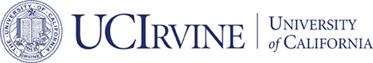# Submitted## From differential equation solvers to accelerated first-order methods for convex optimization

### Hao Luo and Long Chen

#### Submitted

ABSTRACT:

```Convergence analysis of accelerated first-order methods for convex
optimization problems are presented from the point of view of ordinary
differential equation (ODE) solvers. Two resolution ODEs are derived
for accelerated gradient methods.  Numerical discretizations for these
resolution ODEs are considered and its convergence analyses are
established via tailored Lyapunov functions.  The ODE solvers approach
can not only cover existing methods, such as Nesterov's accelerated
gradient method and FISTA, but also produce a large class of new
algorithms that possesses optimal convergence rates. Especially,
linear convergence rate is obtained even for non-strongly convex
function.
```